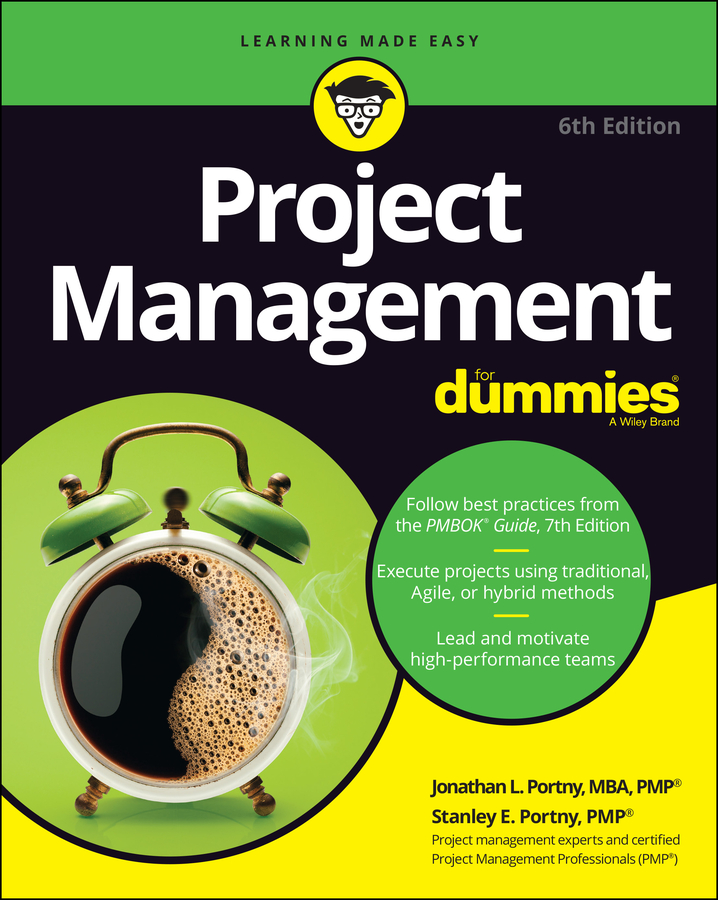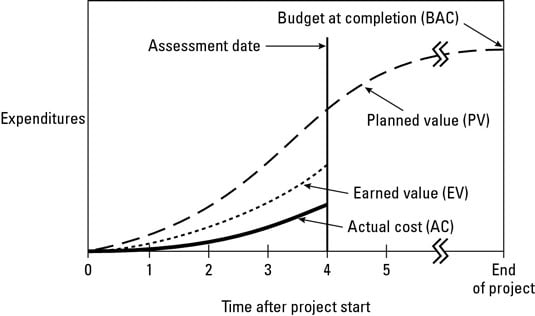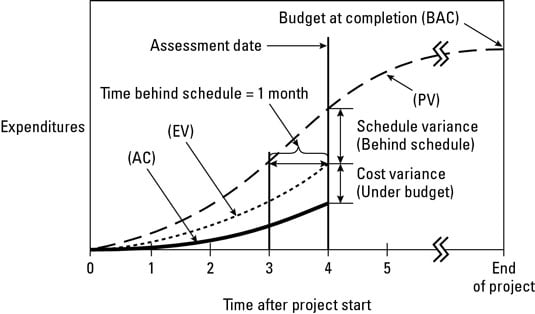##### Project Management For DummiesThe basic premise of earned value management (EVM) is that the value of a piece of work is equal to the amount of funds budgeted to complete it. As part of EVM, you use the following information to assess your schedule and cost performance throughout your project.

• Planned value (PV): The approved budget for the work scheduled to be completed by a specified date; also referred to as the budgeted cost of work scheduled (BCWS). The total PV of a task is equal to the task’s budget at completion (BAC) — the total amount budgeted for the task.

• Earned value (EV): The approved budget for the work actually completed by the specified date; also referred to as the budgeted cost of work performed (BCWP).

• Actual cost (AC): The costs actually incurred for the work completed by the specified date; also referred to as the actual cost of work performed (ACWP).

Monitoring your project’s performance involves determining whether you’re on, ahead of, or behind schedule and on, under, or over budget. But just comparing your actual expenditures with your budget can’t tell you whether you’re on, under, or over budget — which is where EVM comes in.Monitoring planned value, earned value, and actual cost.

To describe your project’s schedule and cost performance with EVM, you use the following indicators:

• Schedule variance (SV): The difference between the amounts budgeted for the work you actually did and for the work you planned to do. The SV shows whether and by how much your work is ahead of or behind your approved schedule.

• Cost variance (CV): The difference between the amount budgeted and the amount actually spent for the work performed. The CV shows whether and by how much you’re under or over your approved budget.

• Schedule performance index (SPI): The ratio of the approved budget for the work performed to the approved budget for the work planned. The SPI reflects the relative amount the project is ahead of or behind schedule, sometimes referred to as the project’s schedule efficiency. You can use the SPI to date to project the schedule performance for the remainder of the task.

• Cost performance index (CPI): The ratio of the approved budget for work performed to what you actually spent for the work. The CPI reflects the relative value of work done compared to the amount paid for it, sometimes referred to as the project’s cost efficiency. You can use the CPI to date to project the cost performance for the remainder of the task.

You can approximate the amount of time you’re behind or ahead of the approved schedule by drawing a line from the intersection of the EV and assessment date lines parallel to the x-axis to the PV line. Doing so suggests that the project being described by the graph is about one month behind schedule.The difference between planned and actual expenditures up to the date of the report is the result of both a schedule delay and cost savings.

Schedule and cost variances and performance indicators are defined mathematically as follows:

• Schedule variance (SV) = Earned value (EV) – Planned value (PV)

• Cost variance (CV) = Earned value (EV) – Actual cost (AC)

• Schedule performance index (SPI) = Earned value (EV) / Planned value (PV)

• Cost performance index (CPI) = Earned value (EV) / Actual cost (AC)

The final step when assessing task performance to date is to update what you expect your total expenditures will be upon task completion. Specifically, you want to determine the following:

• Estimate at completion (EAC): Your estimate today of the total cost of the task

• Estimate to complete (ETC): Your estimate of the amount of funds required to complete all work still remaining to be done on the task

You can use the following two approaches to calculate the EAC:

• Method 1: Assume that the cost performance for the remainder of the task will revert to what was originally budgeted.

EAC = Approved budget for the entire task – Cost variance for the work done to date on the task

= Budget at completion (BAC) + Actual cost (AC) – Earned value (EV)

• Method 2: Assume that the cost performance for the remainder of the task will be the same as what it has been for the work done to date.

EAC = Budget at completion (BAC) / Cumulative cost performance index (CPI)

Whether you use Method 1 or Method 2 to calculate EAC, ETC is determined as follows:

ETC = Budget at completion (BAC) – Actual costs to date (AC)